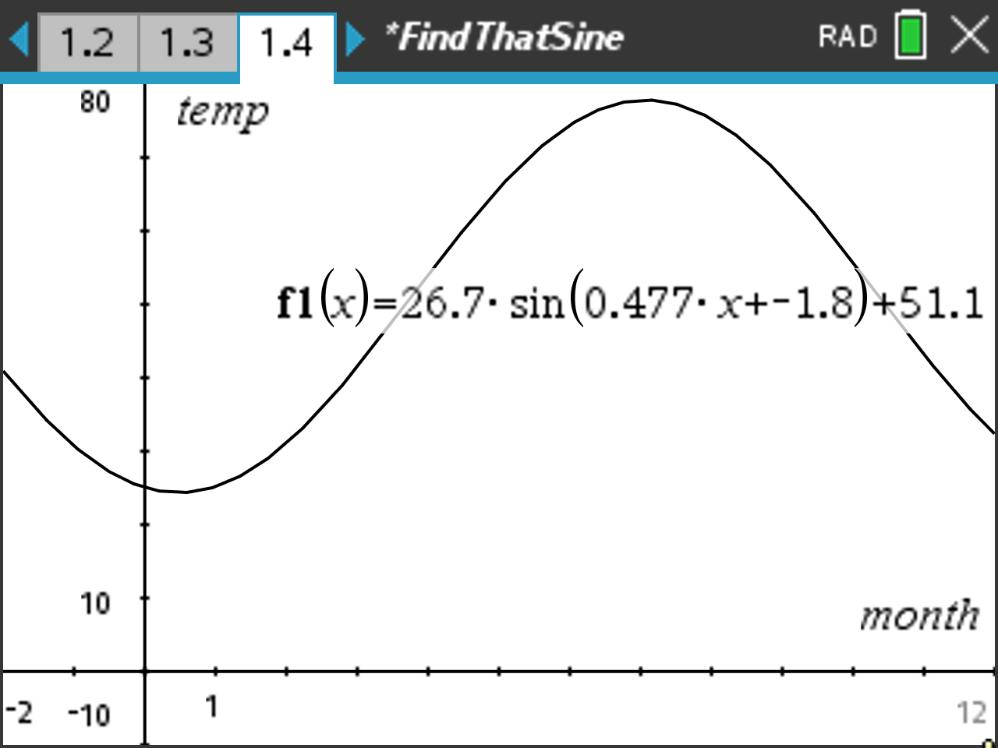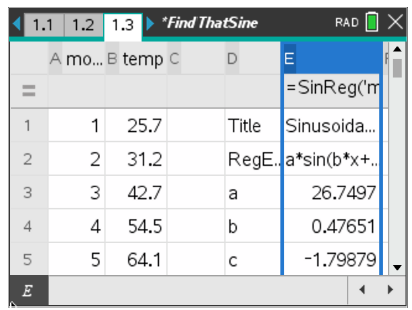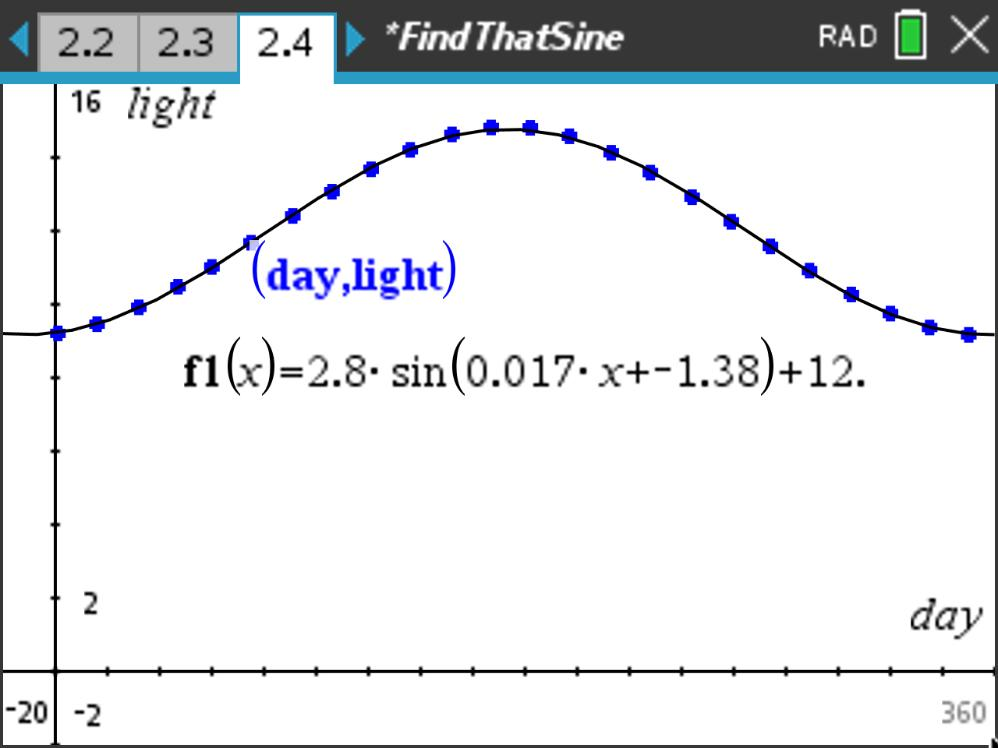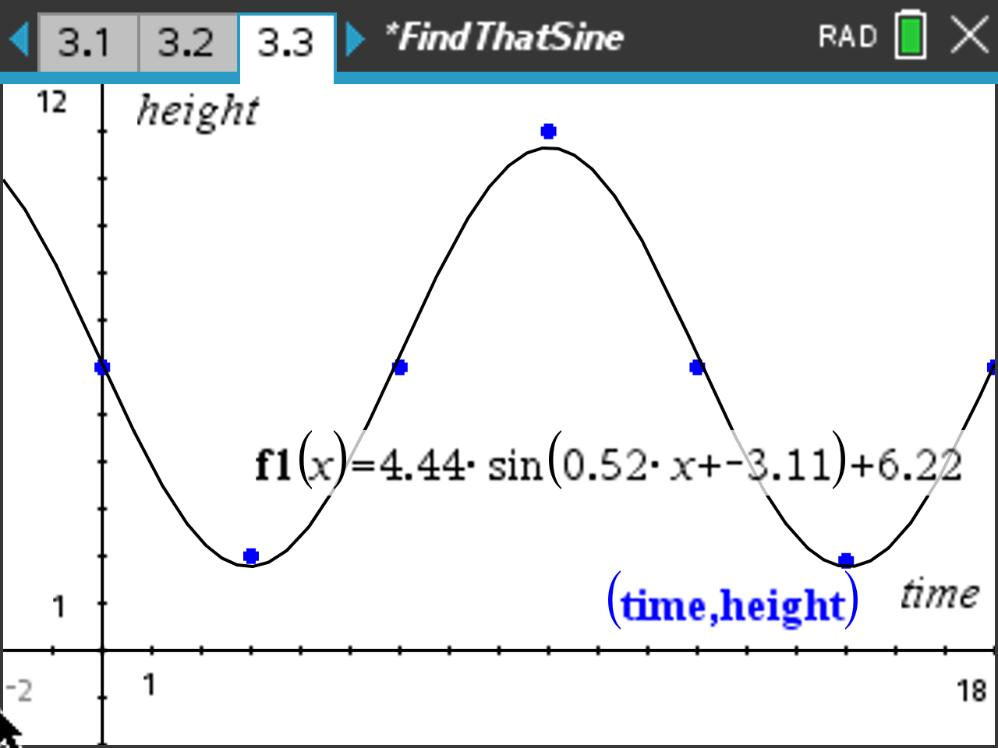### Mathematics lessons for IB® Diploma Programme

Applications and Interpretation | Geometry and Trigonometry## Find That Sine

### Activity Overview

Sinusoidal regression is used to determine equations to model various data sets and the equations are used to make inferences.

## Key Steps## Step 1

Students explore sinusoidal regression by determining and graphing the sine regression equation.

In the first problem, they use data for the average monthly temperature for Kansas City to determine the sine equation that models the data.## Step 2

In Problem 2, students are to find the sinusoidal regression of data that represents the amount of sunlight Kansas City receives from the Sun each day.

Students will then find the minimum and maximum points on the curve. These points will represent the summer and winter solstices.## Step 3

Problem 3 allows students to predict future events using tidal data for the Bay of Fundy.

They can either trace the graph of the equation or they can substitute a value into the graph’s function, example f1(49) to find the height of the water.